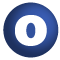scala# PartialFunction

#### object PartialFunction extends AnyRef

A few handy operations which leverage the extra bit of information available in partial functions. Examples:

``` import PartialFunction._ def strangeConditional(other: Any): Boolean = cond(other) {
case x: String if x == "abc" || x == "def"  => true
case x: Int => true
}
def onlyInt(v: Any): Option[Int] = condOpt(v) { case x: Int => x }
```

1. AnyRef
2. Any

### Value Members

1. #### def cond[T](x: T)(pf: PartialFunction[T, Boolean]): Boolean

Creates a Boolean test based on a value and a partial function

Creates a Boolean test based on a value and a partial function. It behaves like a 'match' statement with an implied 'case _ => false' following the supplied cases.

x

the value to test

pf

the partial function

returns

true, iff `x` is in the domain of `pf` and `pf(x) == true`.

2. #### def condOpt[T, U](x: T)(pf: PartialFunction[T, U]): Option[U]

Transforms a PartialFunction[T, U] `pf' into Function1[T, Option[U]] `f' whose result is Some(x) if the argument is in pf's domain and None otherwise, and applies it to the value `x'`

Transforms a PartialFunction[T, U] `pf' into Function1[T, Option[U]] `f' whose result is Some(x) if the argument is in pf's domain and None otherwise, and applies it to the value ```x'. In effect, it is a 'match' statement which wraps all case results in Some(_) and adds 'case _ => None' to the end. ```

x

the value to test

pf

the PartialFunction[T, U]

returns

`Some(pf(x))` if `pf isDefinedAt x`, `None` otherwise.

3. #### def equals(arg0: Any): Boolean

This method is used to compare the receiver object (`this`) with the argument object (`arg0`) for equivalence

This method is used to compare the receiver object (`this`) with the argument object (`arg0`) for equivalence.

The default implementations of this method is an equivalence relation:

• It is reflexive: for any instance `x` of type `Any`, `x.equals(x)` should return `true`.
• It is symmetric: for any instances `x` and `y` of type `Any`, `x.equals(y)` should return `true` if and only if `y.equals(x)` returns `true`.
• It is transitive: for any instances `x`, `y`, and `z` of type `AnyRef` if `x.equals(y)` returns `true` and `y.equals(z)` returns `true`, then `x.equals(z)` should return `true`.

If you override this method, you should verify that your implementation remains an equivalence relation. Additionally, when overriding this method it is often necessary to override `hashCode` to ensure that objects that are "equal" (`o1.equals(o2)` returns `true`) hash to the same `Int` (`o1.hashCode.equals(o2.hashCode)`).

arg0

the object to compare against this object for equality.

returns

`true` if the receiver object is equivalent to the argument; `false` otherwise.

definition classes: AnyRef ⇐ Any
4. #### def hashCode(): Int

Returns a hash code value for the object

Returns a hash code value for the object.

The default hashing algorithm is platform dependent.

Note that it is allowed for two objects to have identical hash codes (`o1.hashCode.equals(o2.hashCode)`) yet not be equal (`o1.equals(o2)` returns `false`). A degenerate implementation could always return `0`. However, it is required that if two objects are equal (`o1.equals(o2)` returns `true`) that they have identical hash codes (`o1.hashCode.equals(o2.hashCode)`). Therefore, when overriding this method, be sure to verify that the behavior is consistent with the `equals` method.

definition classes: AnyRef ⇐ Any
5. #### def toString(): String

Returns a string representation of the object

Returns a string representation of the object.

The default representation is platform dependent.

definition classes: AnyRef ⇐ Any